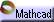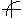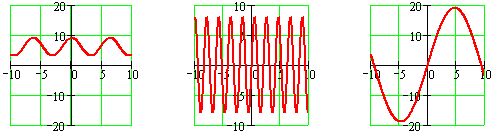Trigonometric Functions Click here to open an associated Mathcad worksheet:Graphic RepresentationGraphs of sine functions all share the same sinusoidal shape: A smoothly varying repetition of peaks and valleys.

Variations among sine graphs are only translations and dilations of the basic  y = sin(x)  graph.

Cosine graphs are simple shifts of sine graphs. The graphs of the other trigonometric functions are ratios of sines and cosines. (See the section on algebraic representations of trigonometric functions.)

The above graphs show just a few of the members of the family of trigonometric functions. To see how these and other trigonometric graphs can be created by tuning the family's parameters, see the associated worksheet.